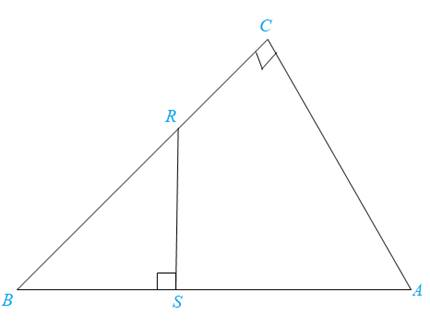Chapter 5.3, Problem 34EElementary Geometry For College St...

7th Edition
Alexander + 2 others
ISBN: 9781337614085

Solutions

Chapter
SectionElementary Geometry For College St...

7th Edition
Alexander + 2 others
ISBN: 9781337614085
Textbook Problem

In Exercise 34, provide a paragraph proof.Given:                       R S ¯ ⊥ A B ¯ , C B ¯ ⊥ A C ¯ Prove:                       Δ B S R ∼ Δ B C ATo determine

To prove:

The statement ΔBSRΔBCA if the sides RS¯ and AB¯ are perpendicular, RS¯AB¯ and the sides CB¯ and AC¯ are perpendicular, CB¯AC¯.

Explanation

Definition:

AA:

If two angles of one triangle are congruent to two angles of another triangle, then the triangles are similar.

Description:

Given that RS¯AB¯ and CB¯AC¯.

The given figure is shown below.

Figure

From the given Figure, it is observed that the sides RS¯ and AB¯ are perpendicular, RS¯AB¯ and the sides CB¯ and

Still sussing out bartleby?

Check out a sample textbook solution.

See a sample solution

The Solution to Your Study Problems

Bartleby provides explanations to thousands of textbook problems written by our experts, many with advanced degrees!

Get Started